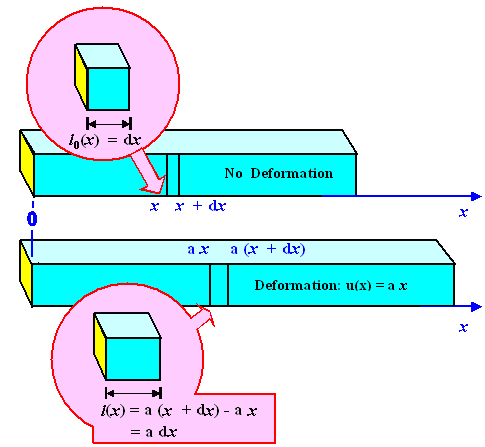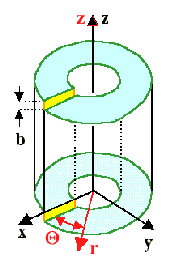# Displacement and StrainIf you take your sword (or any object) and put it somewhere else, you have displaced it. The center of gravity has moved a defined amount, and the object might also have rotated some. If your wife takes your sword (or any other of your objects) and puts it somewhere else, changes are that she misplaced it and you will never find it again. I'm only covering displacements here, since the math of misplacements is highly non-linear and given to screaming fits.
From the viewpoint of the atoms in your sword nothing has changed in either case. They have exactly the same neighbors as before at exactly the same distances. There is no stress and strain, in other words.All that has happened is that you changed the positions of all the atoms within some fixed exterior coordinate system. In an interior coordinate system (pick the three axes of a crystal unit cell, for example) nothing has changed. We conclude:

 A displacement that can be described by a simple coordinate transformation does not produce stress and strain.Stated in other words: Displacement and strain is not the same thing. However, there must be a relation. Displacement changes the position of atoms - and so does strain. Let's go one step further to see how the two relate.We now elongate a longish object elastically in x direction - by a uniaxial tensile test, for example. All the atoms then get displaced but compared to a coordinate transformation by different amounts. In the example the displacement is simply given by a factor x · a as shown below:Displacement for uniaxial deformation.A point that was originally at the position x (upper graph) is now at the position a · x lower graph). Moreover, we certainly now have strain in the specimen. We see that if we look at a differentially small unit cube at the position x (pink inset) . In the starting configuration we assign it the size l0 = dx. At the new position a · x the length of the unit cube is now l(x) = a · (x + dx) - ax = a · dx. There is a strain of (l - l0)/l0 = a - 1.
The general question we need to answer is: How does strain and displacement relate in a general way?First, we need to define displacement in a more rigid way. That is easy. All we need to do is to establish a vector field u(r) that tells us where we find some point originally at r after the displacement has taken place - it's now at r + u(r). Note that different points in the sample may be displaced by different amounts. Note also that simple coordinate transformations are contained in this; they correspond to special u(r) fields.For the simple example of a uniformly elongated rod shown above, we have u(r) = ux(x) = a · x; a is some constant.
In other words, the vector u only has a component in x-direction, which only depends on x as variable. At any point in the rod a little cube then will be deformed into a cuboid - the side in x-direction is somewhat longer than the others.What kind of strain do we have to put on a cube positioned a x, to produce the cuboid?Well, since there is only strain in x-direction, we simply write down the elementary formula for strain

ex  =  l  –  l0
l0
=   ux(x + dx) – ux(x)
dx
=   dux
dxIf we deform uniaxially in all three directions, we get corresponding expressions for ey and ez. Strain, it becomes clear, is simply the derivative of the displacement.But strain is described by a tensor field, displacement by a vector field. Something must be missing.
Yes indeed! So far we have kept the general shape of the specimen. Right angles at the edges are still right angles after the displacement; a cuboid is still a cuboid.Since we also might have displacements that introduce shear, meaning that displacement components in x-direction may depend on y or z, e.g. ux(x, y, z) = a · y, we only get part of the truth if we only consider "straight" derivatives like dux/ dx. There are, after all, also "mixed" or partial derivatives; e.g. ux(x, y, z)/y. What do those derivatives signify?Shear strain, of course. A little less easy to see, perhaps, but there can be no doubt about it. You may want to try to show that for yourself with the simple displacement field given above and the following equations as a guideline for what you are looking for.The relation between a displacement field u(x, y, z) and the strain tensor eij(x, y, z) is given by the following equations:

u(x, y, z)  =  ux(x, y, z)
uy(x, y, z)
uz(x, y, z)

 eij(y, x, z) = exx  exy  exz eyx  eyy  eyz ezx  ezy  ezz æ ç è ö ÷ ø

exx  =  dux
dx
eyy   =  duy
dy
ezz  =  duz
dz

eyz  = æ ç è ö ÷ ø
eyz  = æ ç è ö ÷ ø
eyz  = æ ç è ö ÷ øThis might look a bit frightening but that is misleading. It is often easy to write down the displacement field of some thing, for example around a dislocation. Here is an old picture but this time not with the equations for the stress tensor components but for the displacement associated with a screw dislocation:Essentials of a screw dislocations, allowing to describe the displacement field "by inspection".
uz  =   b · q
2p
=  b
2p
·  tan–1(y/x)  =   b
2p
· arctan (y/x)
Displacement field around a screw dislocation in Cartesian coordinates.
There are only components in z-directionThe elastic displacement field in the dislocated cylinder around a screw dislocation can be found by direct inspection; same thing, albeit a bit more complicated, for an edge dislocation.
First, we note that there are no displacements in the x and y directions, i.e. ux = uy = 0.
Second, in the z-direction, the displacement varies smoothly from 0 to the Burgers vector b as the angle q goes from 0 to 2p. This can be expressed as shown above.
Third, we note that all of this would look much simpler by switching to cylinder coordinates (r, Q, z) (the red system above) but I spare you the math.The basic thing to note is that with a given displacement field, however complex, you only need to do a lot of derivatives to get the strain field! And doing derivatives is trivial (albeit boring), everybody with a halfway decent education can do that. Your computer can do it. There is nothing to it.Bingo! We have strain covered!Numbers and ConcentrationIsotopesBainiteDislocation Science - 1. The BasicsDislocation ScienceScience of Uniaxial DeformationThermal StressScience of Deformation

© H. Föll (Iron, Steel and Swords script)